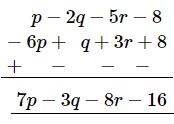# Subtract: −6p + q + 3r + 8 from p − 2q − 5r − 8

Question:

Subtract:
−6p + q + 3r + 8 from p − 2q − 5r − 8

Solution:

Writing the terms of the given expressions (in the same order) in the form of rows with like terms below each other and subtracting column-wise: